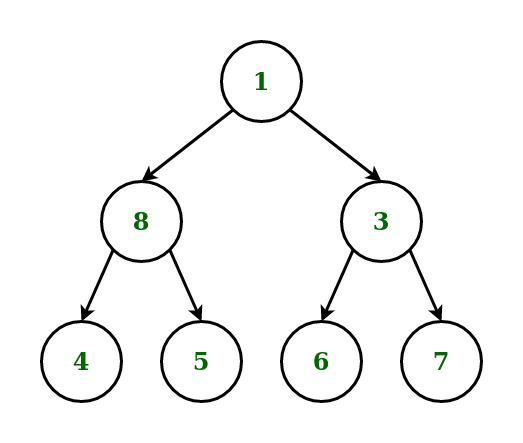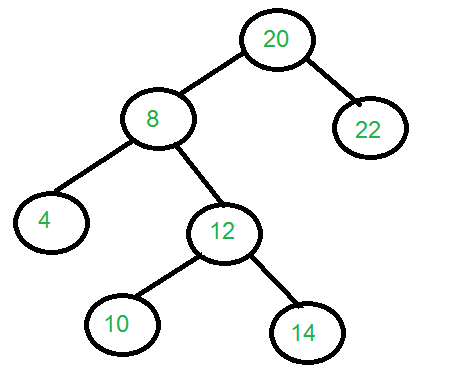# Specific Level Order Traversal of Binary Tree

• Difficulty Level : Hard
• Last Updated : 22 Jun, 2021

Given a Binary Tree, the task is to perform a Specific Level Order Traversal of the tree such that at each level print 1st element then the last element, then 2nd element and 2nd last element, until all elements of that level is printed and so on.

Examples:

Attention reader! Don’t stop learning now. Get hold of all the important DSA concepts with the DSA Self Paced Course at a student-friendly price and become industry ready.  To complete your preparation from learning a language to DS Algo and many more,  please refer Complete Interview Preparation Course.

In case you wish to attend live classes with experts, please refer DSA Live Classes for Working Professionals and Competitive Programming Live for Students.

Input: Below is the given tree:Output: 1 8 3 4 7 5 6
Explanation:
1st level:    1(root)
2nd level:   8(left),    3(right)
3rd level:    4(left),     7(right),    5(left),    6(right)

Input: Below is the given tree:Output: 20 8 22 4 12 10 14
Explanation:
1st level:    20(root)
2nd level:   8(left),    22(right)
3rd level:    4(left),     12(right)
4th level:    10(left),    14(right)

Approach: The idea is to the Level Order Traversal of the given Binary Tree and converts this tree into a Perfect Binary Tree. While traversing if any node whose child Node doesn’t exist then append NULL node as the child node in the queue. Below are the steps:

1. Perform the level order traversal of the given tree.
2. During traversal, if any node whose child Node doesn’t exist then append NULL node as the child node in the queue
3. During traversal for each level do the following:
• Maintain two queues to store the nodes for the two half of each level.
• For the first half of the level store nodes in a left to right manner.
• For the second half of the level store nodes in a right to left manner.
• Iterate the above two queues formed and print in an alternate manner for specific level order traversal.

Below is the implementation of the above approach:

## Java

 `// Java program for the above approach``import` `java.util.*;` `class` `GFG{``    ` `// Node structure``static` `class` `node``{``    ``int` `data;``    ``node left = ``null``;``    ``node right = ``null``;``}`` ` `// Creates and initialize a new node``static` `node newNode(``int` `ch)``{``    ` `    ``// Allocating memory to a new node``    ``node n = ``new` `node();``    ``n.data = ch;``    ``n.left = ``null``;``    ``n.right = ``null``;``    ``return` `n;``}` `// Function to find the height of tree   ``static` `int` `Height(node root)``{``    ``if` `(root == ``null``)``        ``return` `0``;``        ` `    ``return` `1` `+ Math.max(Height(root.left),``                        ``Height(root.right));``}`` ` `// Given a perfect binary tree``// print its node in Specific order``static` `void` `printSpecificLevelOrder(Queue A,``                                    ``Queue B,``                                    ``int` `height)``{``    ``while` `(height != ``0``)``    ``{``        ` `        ``// Get each one front``        ``// element of both queue``        ``node  X = A.poll();``        ``node  Y = B.poll();``        ` `        ``// Check if X exist or not ``        ``if` `(X == ``null``)``        ``{``            ` `            ``// Assume is has and put``            ``// their both child as none``            ``A.add(``null``);``            ``A.add(``null``);``        ``}``        ``else``        ``{``            ` `            ``// print the data and store``            ``// their child first left``            ``// then right``            ``System.out.print(X.data + ``" "``);``            ``A.add(X.left);``            ``A.add(X.right);``        ``}``        ` `        ``// Check Y exist or not   ``        ``if` `(Y == ``null``)``        ``{   ``            ` `            ``// Assume is has and put``            ``// their both child as none``            ``B.add(``null``);``            ``B.add(``null``);``        ``}``        ``else``        ``{``            ` `            ``// Print the data and store their``            ``// child first left then right``            ``System.out.print(Y.data + ``" "``);``            ``B.add(Y.right);``            ``B.add(Y.left);``        ``}``        ` `        ``// Decrease by 1 unit   ``        ``height -= ``1``;     ``    ``}``}` `// Driver Code    ``public` `static` `void` `main (String[] args)``{``    ` `    ``// Given tree``    ``node root = newNode(``1``);``    ``root.left = newNode(``2``);``    ``root.right = newNode(``3``);``    ` `    ``root.left.left = newNode(``4``);``     ``root.left.right = newNode(``5``);``     ``root.right.right = newNode(``7``);``    ` `    ``root.left.left.left = newNode(``8``);``    ``root.left.left.right = newNode(``9``);``    ``root.left.right.left = newNode(``10``);``    ``root.left.right.right = newNode(``11``);``    ``root.right.right.left = newNode(``14``);``    ``root.right.right.right = newNode(``15``);``    ` `    ``root.left.left.left.left = newNode(``16``);``    ``root.left.left.left.right = newNode(``17``);``    ``root.left.left.right.left = newNode(``18``);``    ``root.left.left.right.right = newNode(``19``);``    ``root.left.right.left.left = newNode(``20``);``    ``root.left.right.left.right = newNode(``21``);``    ``root.left.right.right.left = newNode(``22``);``    ``root.left.right.right.right = newNode(``23``);``    ``root.right.right.left.left = newNode(``28``);``    ``root.right.right.left.right = newNode(``29``);``    ``root.right.right.right.left = newNode(``30``);``    ``root.right.right.right.right = newNode(``31``);``    ` `    ``// Initialise Queue``    ``Queue A = ``new` `LinkedList<>();``    ``Queue B = ``new` `LinkedList<>();``    ` `    ``int` `height = ``0``;``    ` `    ``// Check top root manually``    ``if` `(root != ``null``)``    ``{``        ``System.out.print(root.data + ``" "``);``        ` `        ``A.add(root.left);``        ``B.add(root.right);``         ` `        ``height = Height(root);``        ``height = (``int``)Math.pow(``2``, (height - ``1``)) - ``1``;``    ``}``    ` `    ``// Function Call``    ``printSpecificLevelOrder(A, B, height);``}``}` `// This code is contributed by offbeat`

## Python3

 `# Python3 program for the above approach``from` `queue ``import` `Queue` `# A binary tree node``class` `Node:` `    ``# A constructor for making``    ``# a new node``    ``def` `__init__(``self``, key):``        ``self``.data ``=` `key``        ``self``.left ``=` `None``        ``self``.right ``=` `None``    ` `# Function to find the height of tree   ``def` `Height(root):``    ``if` `root ``=``=` `None``:``        ``return` `0``    ``return` `1` `+` `max``(Height(root.left), Height(root.right))`  `# Given a perfect binary tree``# print its node in Specific order``def` `printSpecificLevelOrder(A, B, height):` `    ``while` `height !``=` `0``:` `        ``# Get each one front``        ``# element of both queue``        ``X ``=` `A.get()``        ``Y ``=` `B.get()` `        ``# Check if X exist or not ``        ``if` `X ``=``=` `None``:``            ``# Assume is has and put``            ``# their both child as none``            ``A.put(``None``)``            ``A.put(``None``)``        ``else``:       ``            ``# print the data and store``            ``# their child first left``            ``# then right``            ``print``(X.data, end ``=``" "``)``            ``A.put(X.left)``            ``A.put(X.right)``        ` `        ``# Check Y exist or not   ``        ``if` `Y ``=``=` `None``:      ``            ``# Assume is has and put``            ``# their both child as none``            ``B.put(``None``)``            ``B.put(``None``)   ``        ``else``:``            ``# print the data and store their``            ``# child first left then right``            ``print``(Y.data, end ``=``" "``)``            ``B.put(Y.right)``            ``B.put(Y.left)``        ``# Decrease by 1 unit   ``        ``height``-``=` `1` `      ` `# Driver Code` `# Given Tree``root ``=` `Node(``1``)` `root.left ``=` `Node(``2``)``root.right ``=` `Node(``3``)`  `root.left.left ``=` `Node(``4``)``root.left.right ``=` `Node(``5``)``root.right.right ``=` `Node(``7``)` `root.left.left.left ``=` `Node(``8``)``root.left.left.right ``=` `Node(``9``)``root.left.right.left ``=` `Node(``10``)``root.left.right.right ``=` `Node(``11``)``root.right.right.left ``=` `Node(``14``)``root.right.right.right ``=` `Node(``15``)` `root.left.left.left.left ``=` `Node(``16``)``root.left.left.left.right ``=` `Node(``17``)``root.left.left.right.left ``=` `Node(``18``)``root.left.left.right.right ``=` `Node(``19``)``root.left.right.left.left ``=` `Node(``20``)``root.left.right.left.right ``=` `Node(``21``)``root.left.right.right.left ``=` `Node(``22``)``root.left.right.right.right ``=` `Node(``23``)``root.right.right.left.left ``=` `Node(``28``)``root.right.right.left.right ``=` `Node(``29``)``root.right.right.right.left ``=` `Node(``30``)``root.right.right.right.right ``=` `Node(``31``)` `# Initialise Queue``A ``=` `Queue(``100``)``B ``=` `Queue(``100``)` `# Check top root manually``if` `root !``=` `None``: ``    ``print``(root.data, end ``=``" "``)` `    ``A.put(root.left)``    ``B.put(root.right)``    ` `    ``height ``=` `Height(root)``    ``height ``=` `2``*``*``(height``-``1``)``-``1` `# Function Call``    ``printSpecificLevelOrder(A, B, height)`

## C#

 `// C# program for the above approach``using` `System;``using` `System.Collections.Generic;` `class` `GFG{``    ` `// Node structure``class` `node``{``    ``public` `int` `data;``    ``public` `node left = ``null``;``    ``public` `node right = ``null``;``}`` ` `// Creates and initialize a new node``static` `node newNode(``int` `ch)``{``    ` `    ``// Allocating memory to a new node``    ``node n = ``new` `node();``    ``n.data = ch;``    ``n.left = ``null``;``    ``n.right = ``null``;``    ``return` `n;``}` `// Function to find the height of tree   ``static` `int` `Height(node root)``{``    ``if` `(root == ``null``)``        ``return` `0;``        ` `    ``return` `1 + Math.Max(Height(root.left),``                        ``Height(root.right));``}`` ` `// Given a perfect binary tree``// print its node in Specific order``static` `void` `printSpecificLevelOrder(Queue A,``                                    ``Queue B,``                                    ``int` `height)``{``    ``while` `(height != 0)``    ``{``        ` `        ``// Get each one front``        ``// element of both queue``        ``node X = A.Peek();``        ``A.Dequeue();``        ``node Y = B.Peek();``        ``B.Dequeue();``        ` `        ``// Check if X exist or not ``        ``if` `(X == ``null``)``        ``{``            ` `            ``// Assume is has and put``            ``// their both child as none``            ``A.Enqueue(``null``);``            ``A.Enqueue(``null``);``        ``}``        ``else``        ``{``            ` `            ``// print the data and store``            ``// their child first left``            ``// then right``            ``Console.Write(X.data + ``" "``);``            ``A.Enqueue(X.left);``            ``A.Enqueue(X.right);``        ``}``        ` `        ``// Check Y exist or not   ``        ``if` `(Y == ``null``)``        ``{   ``            ` `            ``// Assume is has and put``            ``// their both child as none``            ``B.Enqueue(``null``);``            ``B.Enqueue(``null``);``        ``}``        ``else``        ``{``            ` `            ``// Print the data and store their``            ``// child first left then right``            ``Console.Write(Y.data + ``" "``);``            ``B.Enqueue(Y.right);``            ``B.Enqueue(Y.left);``        ``}``        ` `        ``// Decrease by 1 unit   ``        ``height -= 1;     ``    ``}``}` `// Driver Code    ``public` `static` `void` `Main()``{``    ` `    ``// Given tree``    ``node root = newNode(1);``    ``root.left = newNode(2);``    ``root.right = newNode(3);``    ` `    ``root.left.left = newNode(4);``     ``root.left.right = newNode(5);``     ``root.right.right = newNode(7);``    ` `    ``root.left.left.left = newNode(8);``    ``root.left.left.right = newNode(9);``    ``root.left.right.left = newNode(10);``    ``root.left.right.right = newNode(11);``    ``root.right.right.left = newNode(14);``    ``root.right.right.right = newNode(15);``    ` `    ``root.left.left.left.left = newNode(16);``    ``root.left.left.left.right = newNode(17);``    ``root.left.left.right.left = newNode(18);``    ``root.left.left.right.right = newNode(19);``    ``root.left.right.left.left = newNode(20);``    ``root.left.right.left.right = newNode(21);``    ``root.left.right.right.left = newNode(22);``    ``root.left.right.right.right = newNode(23);``    ``root.right.right.left.left = newNode(28);``    ``root.right.right.left.right = newNode(29);``    ``root.right.right.right.left = newNode(30);``    ``root.right.right.right.right = newNode(31);``    ` `    ``// Initialise Queue``    ``Queue A = ``new` `Queue();``    ``Queue B = ``new` `Queue();``    ` `    ``int` `height = 0;``    ` `    ``// Check top root manually``    ``if` `(root != ``null``)``    ``{``        ``Console.Write(root.data + ``" "``);``        ` `        ``A.Enqueue(root.left);``        ``B.Enqueue(root.right);``         ` `        ``height = Height(root);``        ``height = (``int``)Math.Pow(2, (height - 1)) - 1;``    ``}``    ` `    ``// Function Call``    ``printSpecificLevelOrder(A, B, height);``}``}` `// This code is contributed by ipg2016107`

## Javascript

 ``
Output:
1 2 3 4 7 5 8 15 9 14 10 11 16 31 17 30 18 29 19 28 20 21 22 23

Time Complexity: O(N), N is the number of nodes
Auxiliary Space: O(N), N is the number of nodes

My Personal Notes arrow_drop_up